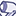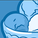# New to Qlik Sense

If you’re new to Qlik Sense, start with this Discussion Board and get up-to-speed quickly.

Announcements
Customer & Partners, DEC. 9, 11 AM ET: Qlik Product & Strategy Roadmap Session: Data Analytics REGISTER NOW
cancel
Showing results for
Did you mean:Contributor III

## Set Analysis- Sum based on date condition

Hi,

I have three fields,

1.Run date- which has the Dates for the past 7 days

2. Sch Remaining Qty- which is the Open order units

3. Material number

Im trying to build a table in which the Open order qty should always show the sum of values for the recent date

I tried with this expression- sum({<[Run Date]-={"\$(=Max([Run Date]))"}>}[Sch Remaining Qty])

But I guess I'm getting all open order values from the previous days.

Can someone help me out to find the Open order units just for the recent date

Thank you,

Vignesh

Labels (4)

• ### Sum Date Ranges

1 Solution

Accepted SolutionsSpecialist

The minus sign "-" in your set analysis is excluding the max run date, which leaves the previous dates.  Try this:

sum({<[Run Date]={"\$(=Max([Run Date]))"}>}[Sch Remaining Qty])

One question: Are you trying to get max([Run Date]) for each Material number?

4 RepliesSpecialist

The minus sign "-" in your set analysis is excluding the max run date, which leaves the previous dates.  Try this:

sum({<[Run Date]={"\$(=Max([Run Date]))"}>}[Sch Remaining Qty])

One question: Are you trying to get max([Run Date]) for each Material number?Contributor III
Author

yes.. Im trying to capture the max([Run Date]) for each material number since date values are there for all material number valuesSpecialist

If [Material Number] is your dimension, you could use the following as a measure:

=FirstSortedValue([Sch Remaining Qty],-[Run Date])

to give you the most recent [Sch Remaining Qty] for each [Material Number].Contributor III
Author

@GaryGiles  Thank you so much.  Would using this expression-sum({<[Run Date]={"\$(=Max([Run Date]))"}>}[Sch Remaining Qty]) still gives me the correct sum of Sch Remaining Qty based on latest Run date right?

Also one question- How to modify the above sum expression if the Run Date is included on the filter pane- meaning if I change the dates on the Filter pane- expression should modify to give me the sum of values based on the filtered date otherwise it should give me the sum of values based on recent date. Is it possible?Tags
Community Browser